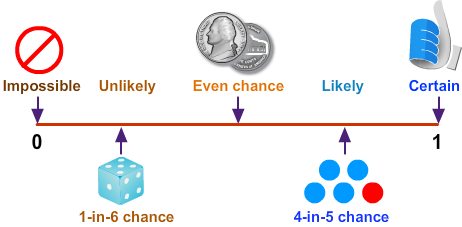# Probability

Print Rate 0 stars
Lesson size:
Message preview:
Someone you know has shared lesson with you:

To play this lesson, click on the link below:

https://www.turtlediary.com/lesson/probability.html

Hope you have a good experience with this site and recommend to your friends too.

Login to rate activities and track progress.
Login to rate activities and track progress.
Probability is simply the likelihood of an event happening.It can be expressed in the following ways:

• Equal Probability
• Likely Probability
• Unlikely Probability
• Certain Probability
• Impossible Probability

## Equal Probability

### Example 1There are 2 pens in a bag: one red and one blue. Since there is one of each, the likelihood of picking a red or a blue pen is equal, therefore the probability is equal.

The probability of picking blue pen is
1
2
.
And the probability of picking red pen is
1
2
.

### Example 2There are 2 balls in a bag: one purple and one blue. Since there is one of each, the likelihood of picking a purple or a blue ball is equal, therefore the probability is equal.

The probability of picking purple ball is
1
2
.
And the probability of picking blue ball is
1
2
.

## Likely Probability

### Example 1Here we have 3 pens: 2 red, 1 blue. Since we have more red pens, the probability of picking out a red is higher than (likely) that of picking out a blue.

The probability of picking out a red pen is
2
3
.

### Example 2Now the probability of picking a red ball would be higher than (likely) that of picking a blue or a green ball, since there are more red than any other colored ball in the set. Notice that the total number in the set changes as well. The probability of picking out a red ball would be 3 out of 6 or
3
6
.

## Unlikely Probability

### Example 1It's less likely (unlikely) that you would pick out a blue pen, since there are more red pens than blue ones. The probability of picking out blue would be written as 1 out of 3, or
1
3
.

### Example 2Here we have 5 balls: one blue, two red, and two green. You ask someone to pick one. The likelihood that they will pick a blue ball is lower than (unlikely) the likelihood that they will pick a red or a green ball because there is only one blue while there are two red and two green. The probability of picking out a blue ball would be written as 1 out of 5 or
1
5
.

## Certain Probability

The word certain means that an event will definitely happen without a doubt.

### Example 1If we had a set of all blue pens, we could say that it is certain that we would pick out a blue pen from this set, since this is the only option.

### Example 2If we had a set of all red balls, we could say that it is certain that we would pick out a red ball from this set, since this is the only option.

## Impossible Probability

Impossible means that there's no way that event will happen.

### Example 1We can say that it is impossible that a green pen will be picked out because there are no green pens in this set.

### Example 2Here, we can say that it is impossible that a black ball will be picked out because there are no black balls in this set.

## Practice with a die

Jenny rolls a six-sided die. The six sides of the die have the numbers 1 - 6 written on them. For each of the following, state if the probability is certain, probable, unlikely, or impossible.

Probable Probability

Jenny will roll a number greater than 1:

There are 6 numbers that might be rolled. 5 of them are greater than 1. Because most of the numbers are greater than 1, it is probable that Jenny will roll a number greater than 1.

Certain Probability

Jenny will roll a number less than 10:

There are 6 numbers on the die. All 6 of them are less than 10. Because all of the numbers are less than 10, it is certain that Jenny will roll a number less than 10.

Impossible Probability

Jenny will roll a number greater than 6:

There are 6 numbers on the die. None of the numbers are greater than 6. Because none of them are greater than 6, it is impossible that Jenny will roll a number greater than 6.

Unlikely Probability

Jenny will roll a number less than 2:

There are 6 numbers on the die. Only 1 of those numbers is less than 2. Because most of the numbers are NOT less than 2, it is unlikely that Jenny will choose a number that is less than 2.

## Probability

• Probability is simply the likelihood of an event happening.
• It can be expressed in the following ways:
• Equal Probability
• Likely Probability
• Unlikely Probability
• Certain Probability
• Impossible Probability
Become premium member to get unlimited access.